# B-Tree Insert without aggressive splitting

B-Tree Insert without aggressive splitting

This algorithm for insertion takes an entry, finds the leaf node where it belongs, and inserts it there. We recursively insert the entry by calling the insert algorithm on the appropriate child node. This procedure results in going down to the leaf node where the entry belongs, placing the entry there, and returning all the way back to the root node.

Sometimes a node is full, i.e. it contains 2*t – 1 entries where t is the minimum degree. In such cases the node must be split. In such case one key becomes a parent and a new node is created. We first insert the new key, making total keys as 2*t. We keep the first t entries in original node, transfer last (t-1) entries to new node and set the (t+1)th node as parent of these nodes. If the node being split is a non child node then we also have to split the child pointers. A node having 2*t keys has 2*t + 1 child pointers. The first (t+1) pointers are kept in original node and remaining t pointers goes to new node.

This algorithm splits a node only when it is necessary. We first recursively call insert for appropriate child of node (in case of non-leaf node) or insert it into node (for leaf node). If the node is full, we split it, storing new child entry in newEntry and new parent key in val. These values are then inserted into the parent, which recursively splits itself in case it is also full.

Example:

We insert numbers 1 – 5 in tree. The tree becomes: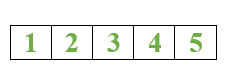Then we insert 6, the node is full. Hence it is split into two nodes making 4 as parent.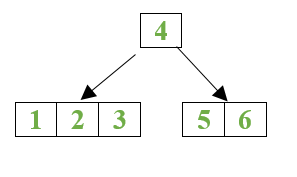We insert numbers 7 – 16, the tree becomes: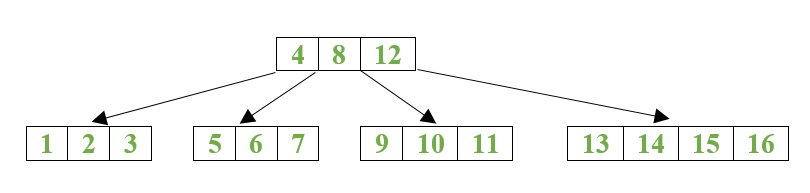We insert 22 – 30, the tree becomes: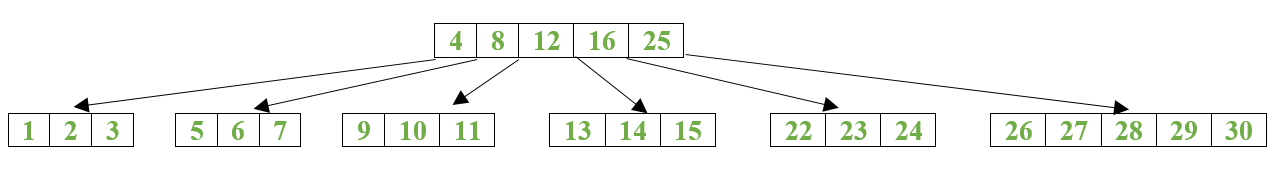Note that now the root is full. If we insert 17 now, the root is not split as the leaf node in which 17 was inserted didn’t split. If we were following aggressive splitting, the root would have been split before we went to leaf node.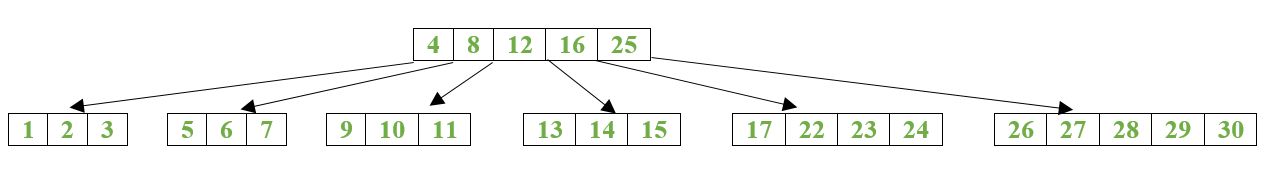But if we insert 31, the leaf node splits, which recursively adds new entry to root. But as root is full, it needs to be split. The tree now becomes.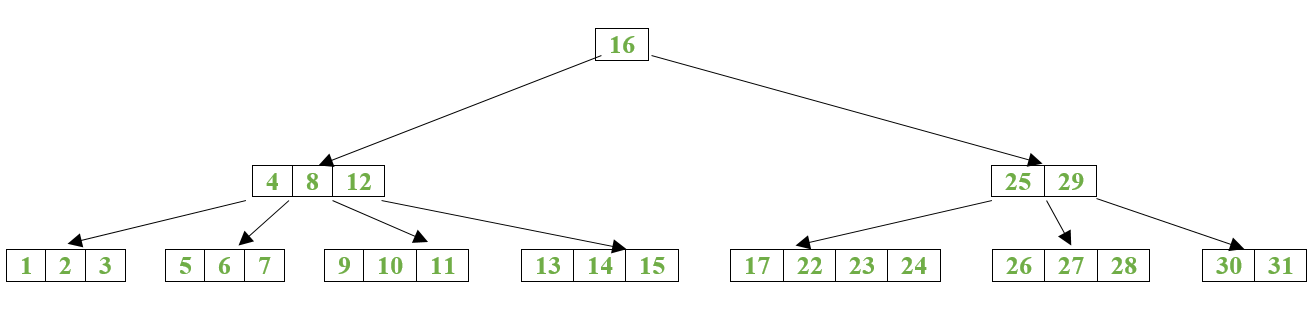Below is the implementation of the above approach:

 `// C++ implementation of the approach ` `#include ` `#include ` `using` `namespace` `std; ` ` `  `class` `BTreeNode { ` ` `  `    ``// Vector of keys ` `    ``vector<``int``> keys; ` ` `  `    ``// Minimum degree ` `    ``int` `t; ` ` `  `    ``// Vector of child pointers ` `    ``vector C; ` ` `  `    ``// Is true when node is leaf, else false ` `    ``bool` `leaf; ` ` `  `public``: ` `    ``// Constructor ` `    ``BTreeNode(``int` `t, ``bool` `leaf); ` ` `  `    ``// Traversing the node and print its content ` `    ``// with tab number of tabs before ` `    ``void` `traverse(``int` `tab); ` ` `  `    ``// Insert key into given node. If child is split, we ` `    ``// have to insert *val entry into keys vector and ` `    ``// newEntry pointer into C vector of this node ` `    ``void` `insert(``int` `key, ``int``* val, ` `                ``BTreeNode*& newEntry); ` ` `  `    ``// Split this node and store the new parent value in ` `    ``// *val and new node pointer in newEntry ` `    ``void` `split(``int``* val, BTreeNode*& newEntry); ` ` `  `    ``// Returns true if node is full ` `    ``bool` `isFull(); ` ` `  `    ``// Makes new root, setting current root as its child ` `    ``BTreeNode* makeNewRoot(``int` `val, BTreeNode* newEntry); ` `}; ` ` `  `bool` `BTreeNode::isFull() ` `{ ` `    ``// returns true if node is full ` `    ``return` `(``this``->keys.size() == 2 * t - 1); ` `} ` ` `  `BTreeNode::BTreeNode(``int` `t, ``bool` `leaf) ` `{ ` `    ``// Constructor to set value of t and leaf ` `    ``this``->t = t; ` `    ``this``->leaf = leaf; ` `} ` ` `  `// Function to print the nodes of B-Tree ` `void` `BTreeNode::traverse(``int` `tab) ` `{ ` `    ``int` `i; ` `    ``string s; ` ` `  `    ``// Print 'tab' number of tabs ` `    ``for` `(``int` `j = 0; j < tab; j++) { ` `        ``s += ``'\t'``; ` `    ``} ` `    ``for` `(i = 0; i < keys.size(); i++) { ` ` `  `        ``// If this is not leaf, then before printing key[i] ` `        ``// traverse the subtree rooted with child C[i] ` `        ``if` `(leaf == ``false``) ` `            ``C[i]->traverse(tab + 1); ` `        ``cout << s << keys[i] << endl; ` `    ``} ` ` `  `    ``// Print the subtree rooted with last child ` `    ``if` `(leaf == ``false``) { ` `        ``C[i]->traverse(tab + 1); ` `    ``} ` `} ` ` `  `// Function to split the current node and store the new ` `// parent value is *val and new child pointer in &newEntry ` `// called only for splitting non-leaf node ` `void` `BTreeNode::split(``int``* val, BTreeNode*& newEntry) ` `{ ` ` `  `    ``// Create new non leaf node ` `    ``newEntry = ``new` `BTreeNode(t, ``false``); ` ` `  `    ``//(t+1)th becomes parent ` `    ``*val = ``this``->keys[t]; ` ` `  `    ``// Last (t-1) entries will go to new node ` `    ``for` `(``int` `i = t + 1; i < 2 * t; i++) { ` `        ``newEntry->keys.push_back(``this``->keys[i]); ` `    ``} ` ` `  `    ``// This node stores first t entries ` `    ``this``->keys.resize(t); ` ` `  `    ``// Last t entries will go to new node ` `    ``for` `(``int` `i = t + 1; i <= 2 * t; i++) { ` `        ``newEntry->C.push_back(``this``->C[i]); ` `    ``} ` ` `  `    ``// This node stores first (t+1) entries ` `    ``this``->C.resize(t + 1); ` `} ` ` `  `// Function to insert a new key in given node. ` `// If child of this node is split, we have to insert *val ` `// into keys vector and newEntry pointer into C vector ` `void` `BTreeNode::insert(``int` `new_key, ``int``* val, ` `                       ``BTreeNode*& newEntry) ` `{ ` ` `  `    ``// Non leaf node ` `    ``if` `(leaf == ``false``) { ` `        ``int` `i = 0; ` ` `  `        ``// Find first key greater than new_key ` `        ``while` `(i < keys.size() && new_key > keys[i]) ` `            ``i++; ` ` `  `        ``// We have to insert new_key into left child of ` `        ``// Node with index i ` `        ``C[i]->insert(new_key, val, newEntry); ` ` `  `        ``// No split was done ` `        ``if` `(newEntry == NULL) ` `            ``return``; ` `        ``if` `(keys.size() < 2 * t - 1) { ` ` `  `            ``// This node can accomodate a new key ` `            ``// and child pointer entry ` `            ``// Insert *val into key vector ` `            ``keys.insert(keys.begin() + i, *val); ` ` `  `            ``// Insert newEntry into C vector ` `            ``C.insert(C.begin() + i + 1, newEntry); ` ` `  `            ``// As this node was not split, set newEntry ` `            ``// to NULL ` `            ``newEntry = NULL; ` `        ``} ` `        ``else` `{ ` ` `  `            ``// Insert *val and newentry ` `            ``keys.insert(keys.begin() + i, *val); ` `            ``C.insert(C.begin() + i + 1, newEntry); ` ` `  `            ``// Current node has 2*t keys, so split it ` `            ``split(val, newEntry); ` `        ``} ` `    ``} ` `    ``else` `{ ` ` `  `        ``// Insert new_key in this node ` `        ``vector<``int``>::iterator it; ` ` `  `        ``// Find correct position ` `        ``it = lower_bound(keys.begin(), keys.end(), ` `                         ``new_key); ` ` `  `        ``// Insert in correct position ` `        ``keys.insert(it, new_key); ` ` `  `        ``// If node is full ` `        ``if` `(keys.size() == 2 * t) { ` ` `  `            ``// Create new node ` `            ``newEntry = ``new` `BTreeNode(t, ``true``); ` ` `  `            ``// Set (t+1)th key as parent ` `            ``*val = ``this``->keys[t]; ` ` `  `            ``// Insert last (t-1) keys into new node ` `            ``for` `(``int` `i = t + 1; i < 2 * t; i++) { ` `                ``newEntry->keys.push_back(``this``->keys[i]); ` `            ``} ` ` `  `            ``// This node stores first t keys ` `            ``this``->keys.resize(t); ` `        ``} ` `    ``} ` `} ` ` `  `// Function to create a new root ` `// setting current node as its child ` `BTreeNode* BTreeNode::makeNewRoot(``int` `val, BTreeNode* newEntry) ` `{ ` `    ``// Create new root ` `    ``BTreeNode* root = ``new` `BTreeNode(t, ``false``); ` ` `  `    ``// Stores keys value ` `    ``root->keys.push_back(val); ` ` `  `    ``// Push child pointers ` `    ``root->C.push_back(``this``); ` `    ``root->C.push_back(newEntry); ` `    ``return` `root; ` `} ` ` `  `class` `BTree { ` ` `  `    ``// Root of B-Tree ` `    ``BTreeNode* root; ` ` `  `    ``// Minimum degree ` `    ``int` `t; ` ` `  `public``: ` `    ``// Constructor ` `    ``BTree(``int` `t); ` ` `  `    ``// Insert key ` `    ``void` `insert(``int` `key); ` ` `  `    ``// Display the tree ` `    ``void` `display(); ` `}; ` ` `  `// Function to create a new BTree with ` `// minimum degree t ` `BTree::BTree(``int` `t) ` `{ ` `    ``root = ``new` `BTreeNode(t, ``true``); ` `} ` ` `  `// Function to insert a node in the B-Tree ` `void` `BTree::insert(``int` `key) ` `{ ` `    ``BTreeNode* newEntry = NULL; ` `    ``int` `val = 0; ` ` `  `    ``// Insert in B-Tree ` `    ``root->insert(key, &val, newEntry); ` ` `  `    ``// If newEntry is not Null then root needs to be ` `    ``// split. Create new root ` `    ``if` `(newEntry != NULL) { ` `        ``root = root->makeNewRoot(val, newEntry); ` `    ``} ` `} ` ` `  `// Prints BTree ` `void` `BTree::display() ` `{ ` `    ``root->traverse(0); ` `} ` ` `  `// Driver code ` `int` `main() ` `{ ` ` `  `    ``// Create B-Tree ` `    ``BTree* tree = ``new` `BTree(3); ` `    ``cout << ``"After inserting 1 and 2"` `<< endl; ` `    ``tree->insert(1); ` `    ``tree->insert(2); ` `    ``tree->display(); ` ` `  `    ``cout << ``"After inserting 5 and 6"` `<< endl; ` `    ``tree->insert(5); ` `    ``tree->insert(6); ` `    ``tree->display(); ` ` `  `    ``cout << ``"After inserting 3 and 4"` `<< endl; ` `    ``tree->insert(3); ` `    ``tree->insert(4); ` `    ``tree->display(); ` ` `  `    ``return` `0; ` `} `

Output:

```After inserting 1 and 2
1
2
After inserting 5 and 6
1
2
5
6
After inserting 3 and 4
1
2
3
4
5
6
```

My Personal Notes arrow_drop_upCheck out this Author's contributed articles.

If you like GeeksforGeeks and would like to contribute, you can also write an article using contribute.geeksforgeeks.org or mail your article to contribute@geeksforgeeks.org. See your article appearing on the GeeksforGeeks main page and help other Geeks.

Please Improve this article if you find anything incorrect by clicking on the "Improve Article" button below.

Article Tags :
Practice Tags :

2

Please write to us at contribute@geeksforgeeks.org to report any issue with the above content.